# NCERT Exemplar Solutions for Class 9 Maths Chapter 1 - Number Systems

## NCERT Exemplar Solutions Class 9 Maths Chapter 1 – Free PDF Download

NCERT Exemplar Class 9 Maths Chapter 1 Number System is provided here for students to prepare for exams. These exemplars problems and solutions are designed by experts in accordance with CBSE Syllabus for 9th standard, which covers the following topics of Number System given below:

• Rational Numbers and Irrational numbers
• Finding rational numbers between two given numbers
• Locating irrational numbers in a number line
• Real Numbers and their Decimal Expansions is terminating or non-terminating, recurring or non-recurring.
• Finding irrational numbers between two given numbers
• Operations performed on real numbers
• Rationalizing the denominator
• Laws of Exponents for Real Numbers

To facilitate easy learning and help students understand the concepts discussed in Chapter 1, free NCERT Exemplars are provided here which can be further downloaded in the form of a PDF. Students can use these materials as a reference tool for studying as well as practicing sums. The exemplars also contain solved questions relevant to the exercise problems present in the NCERT textbook.

## Download PDF of NCERT Exemplar Solutions for Class 9 Maths Chapter 1 – Number Systems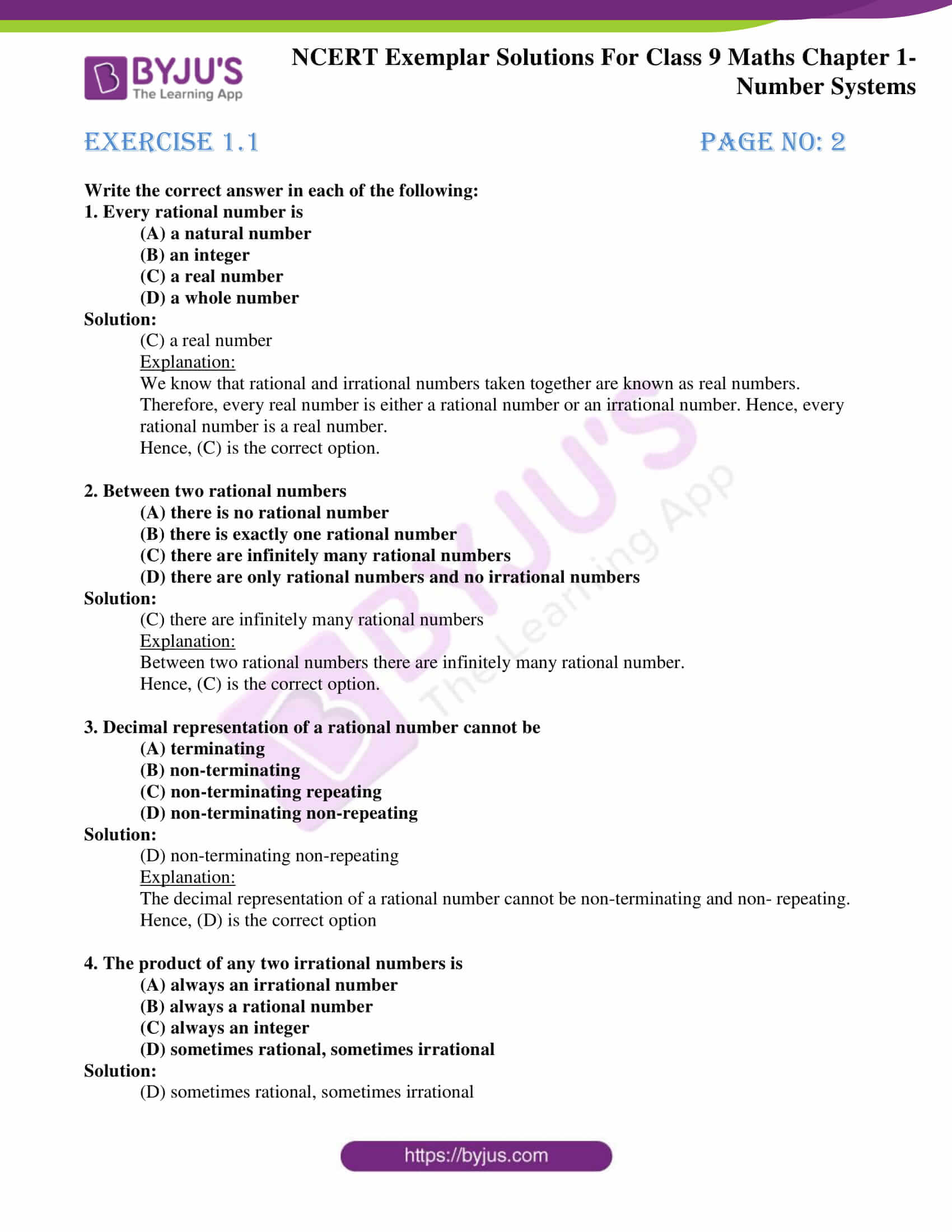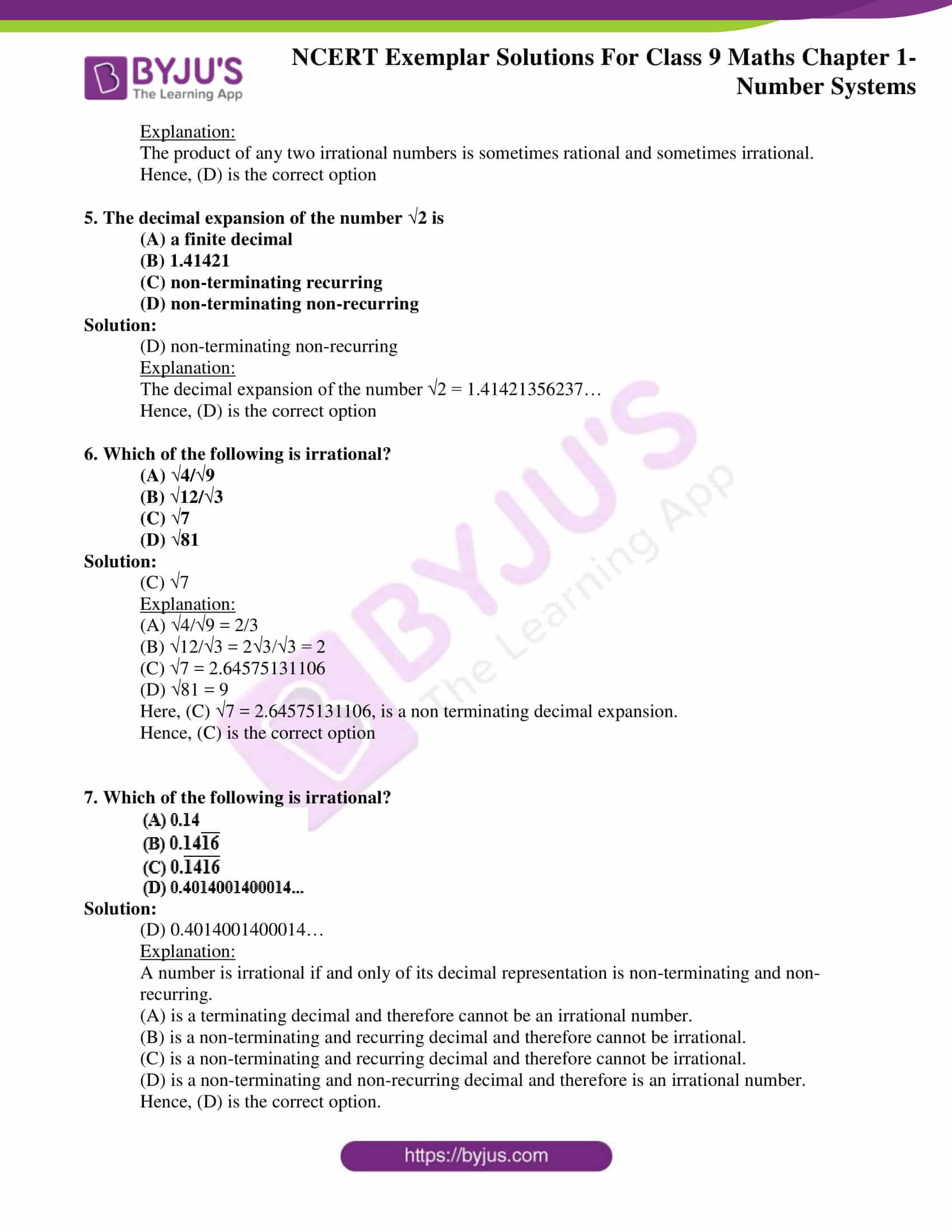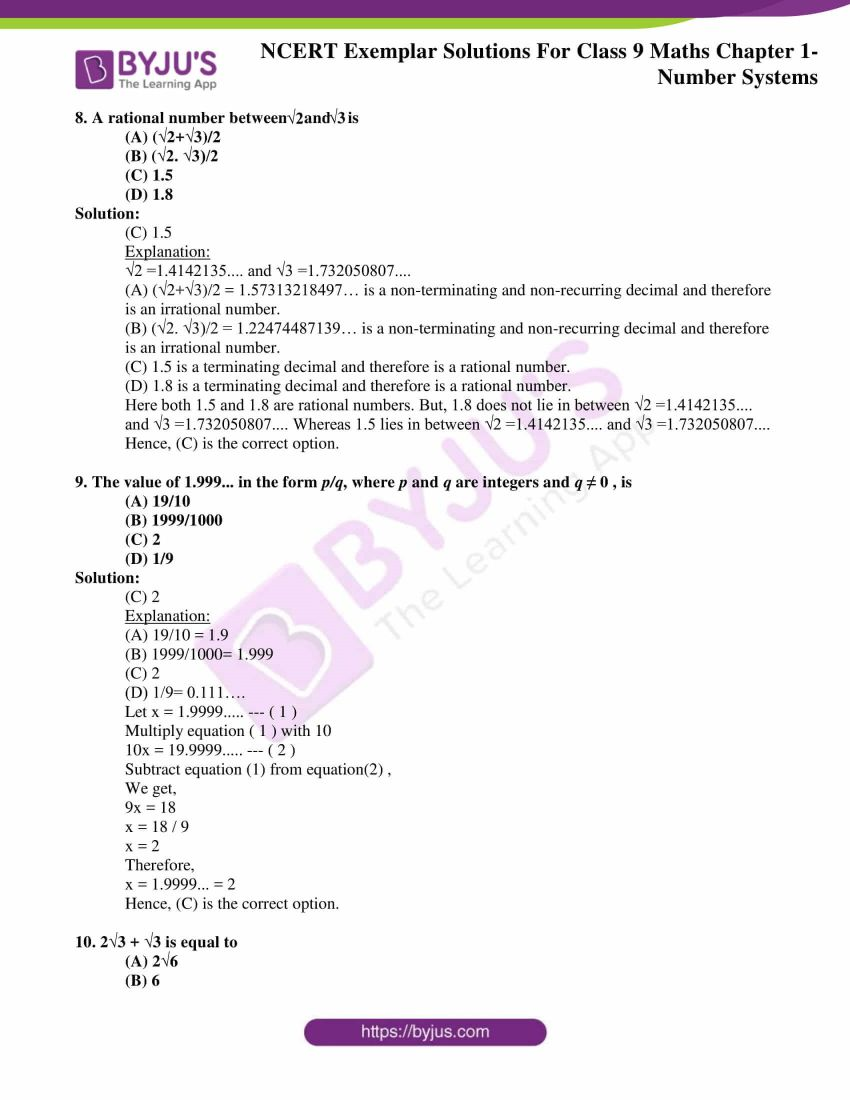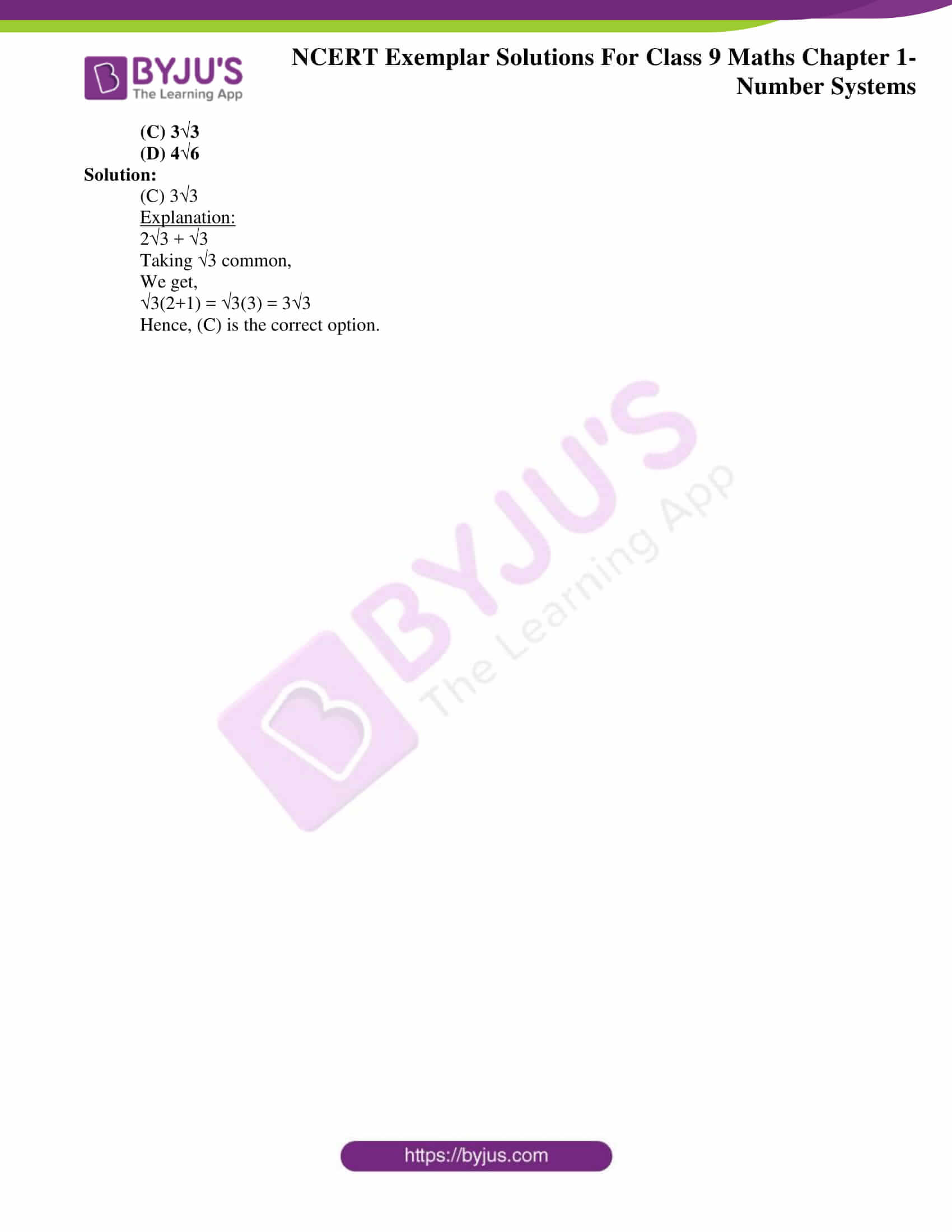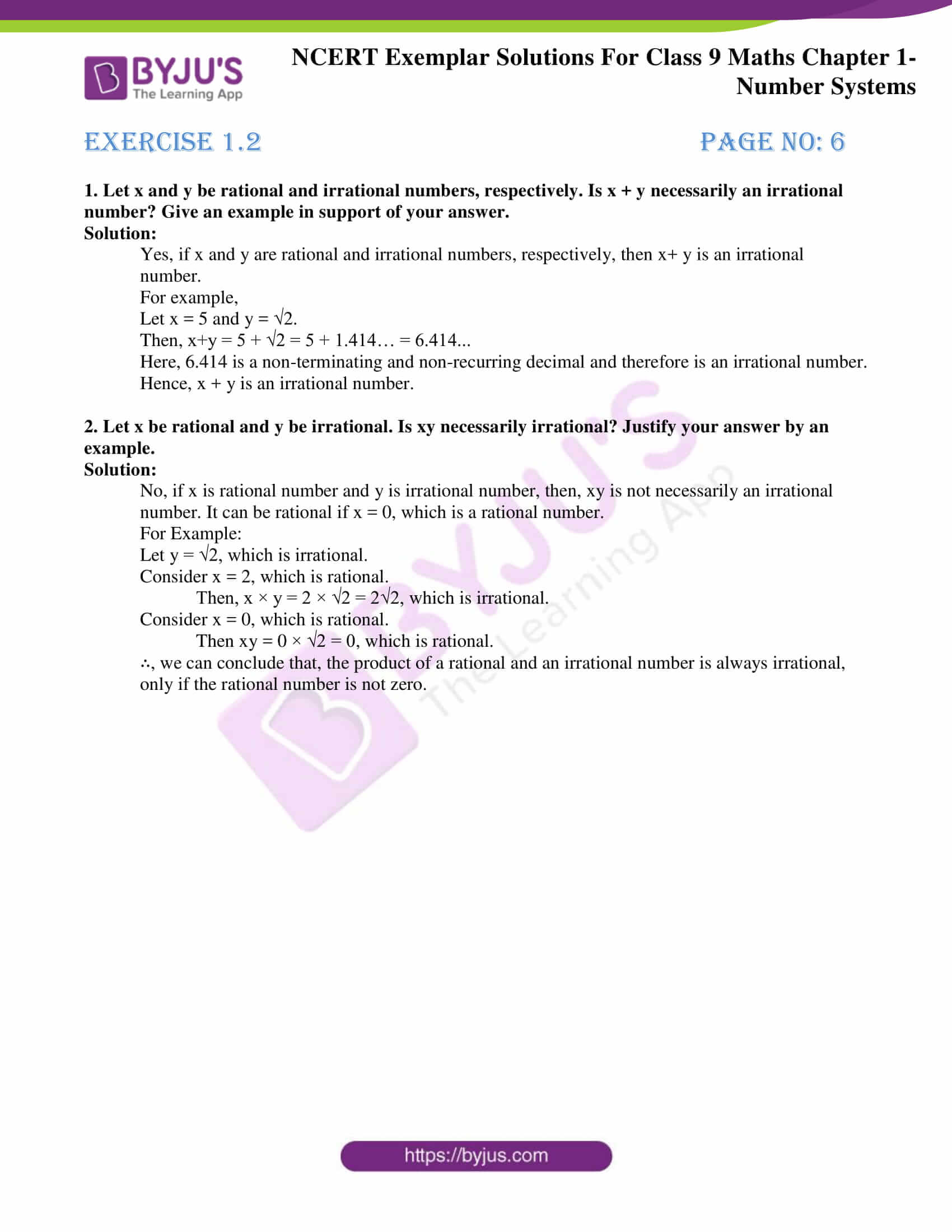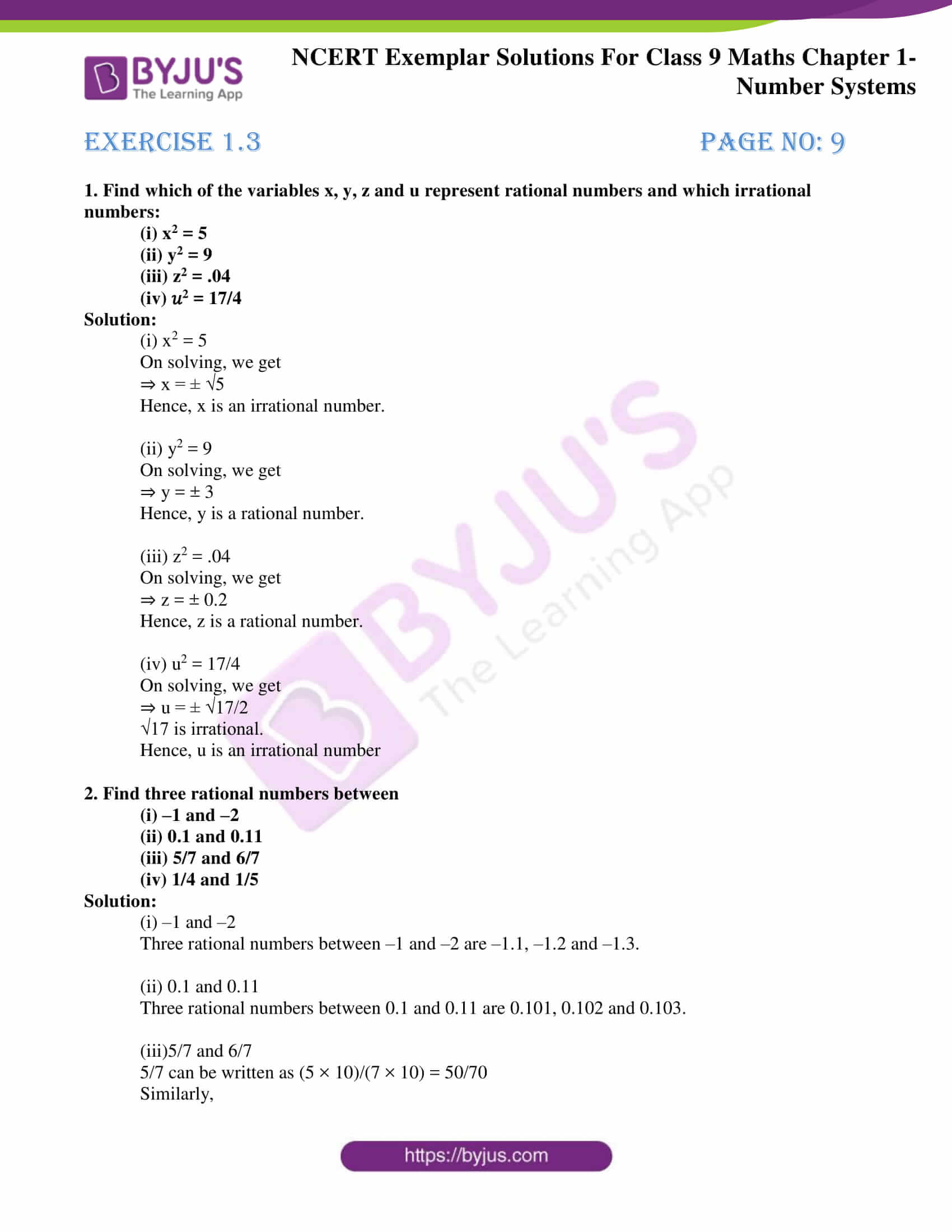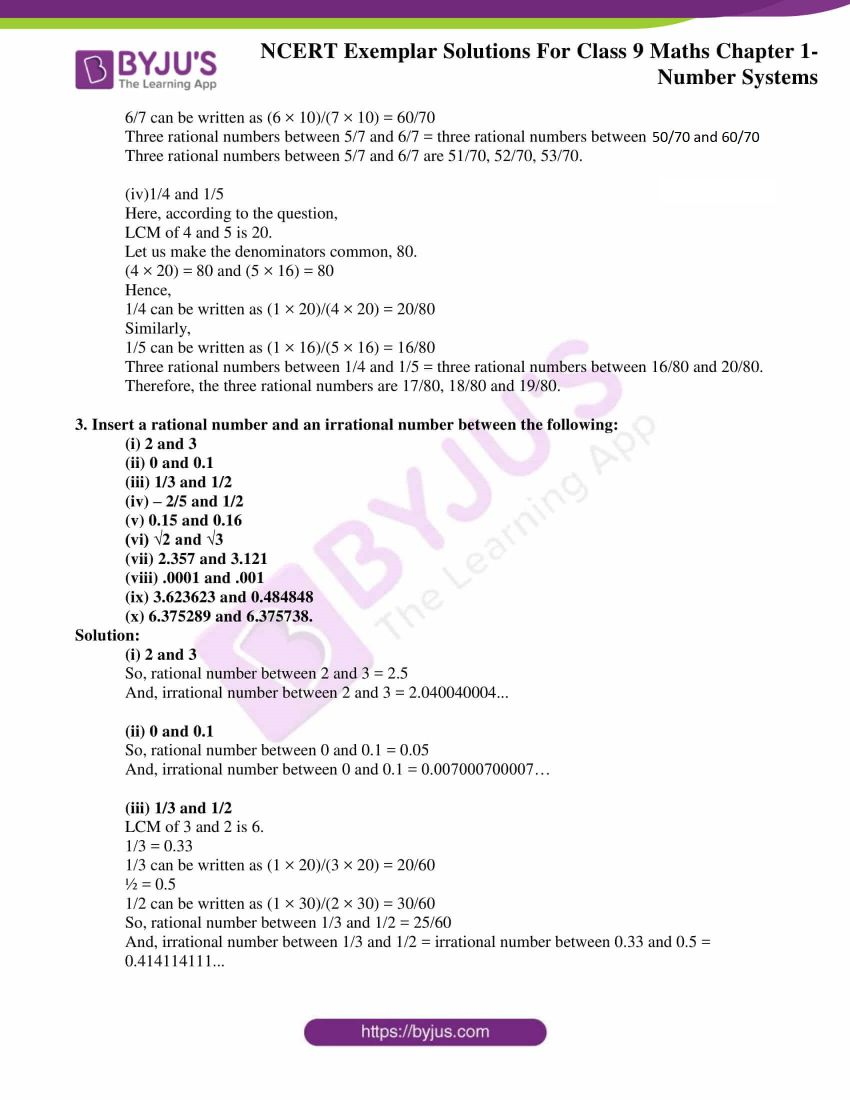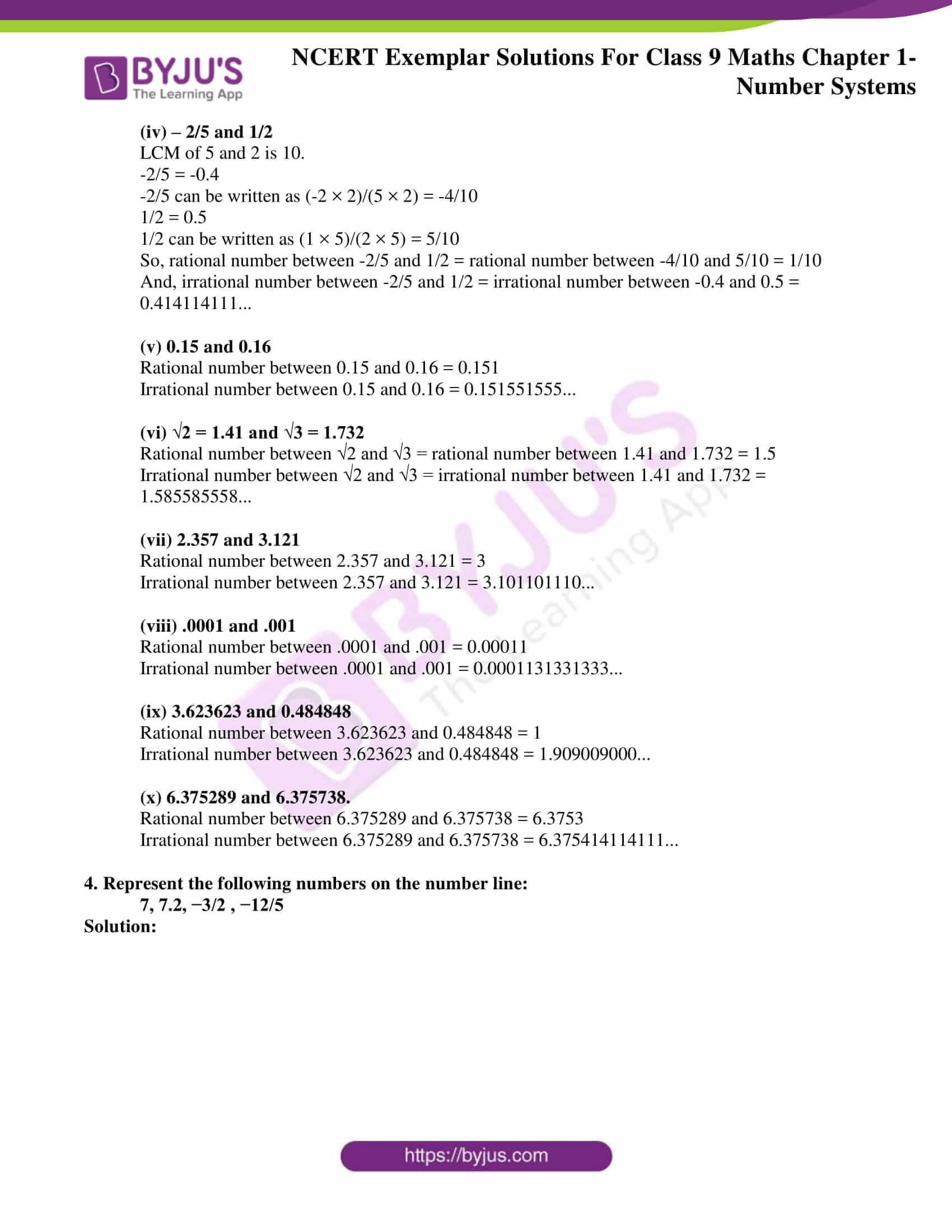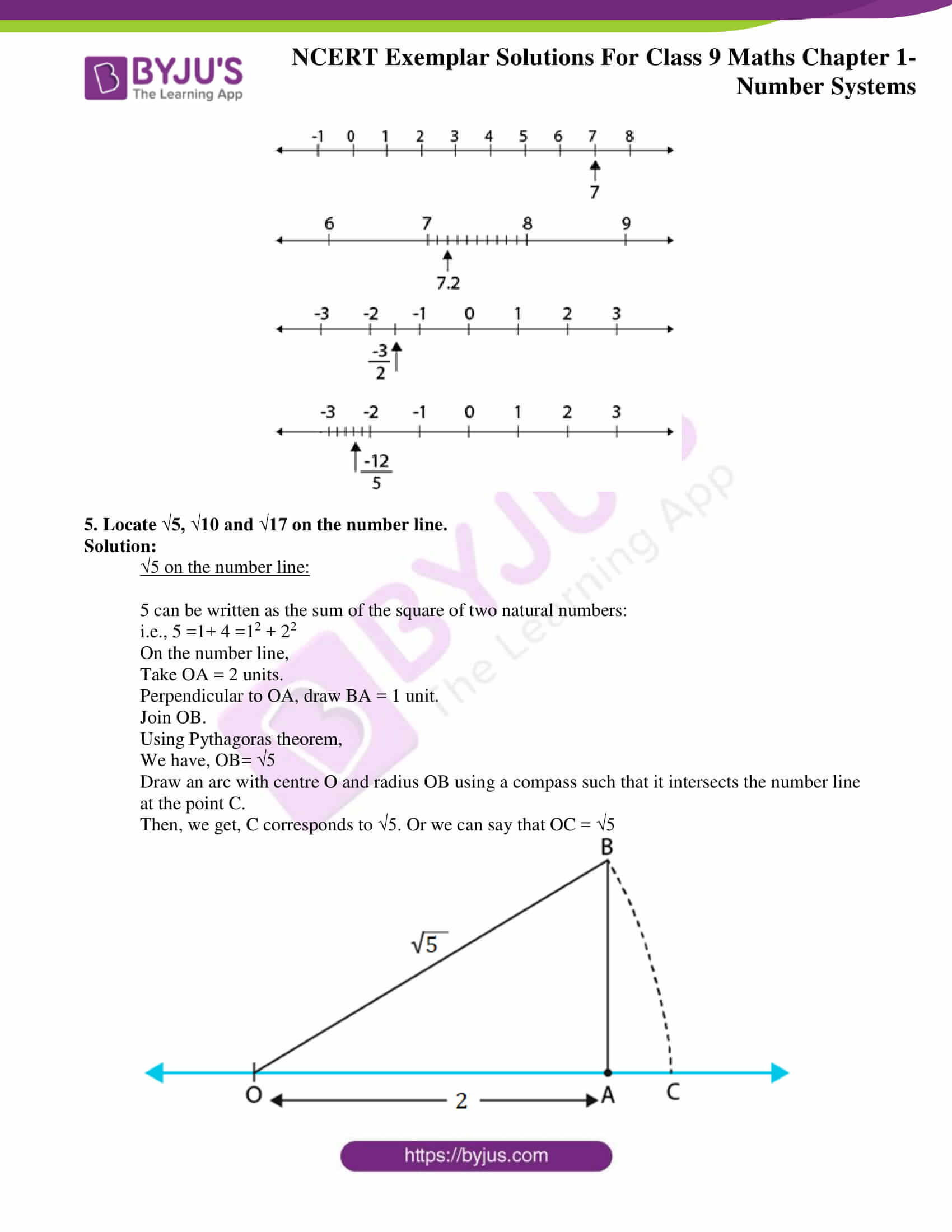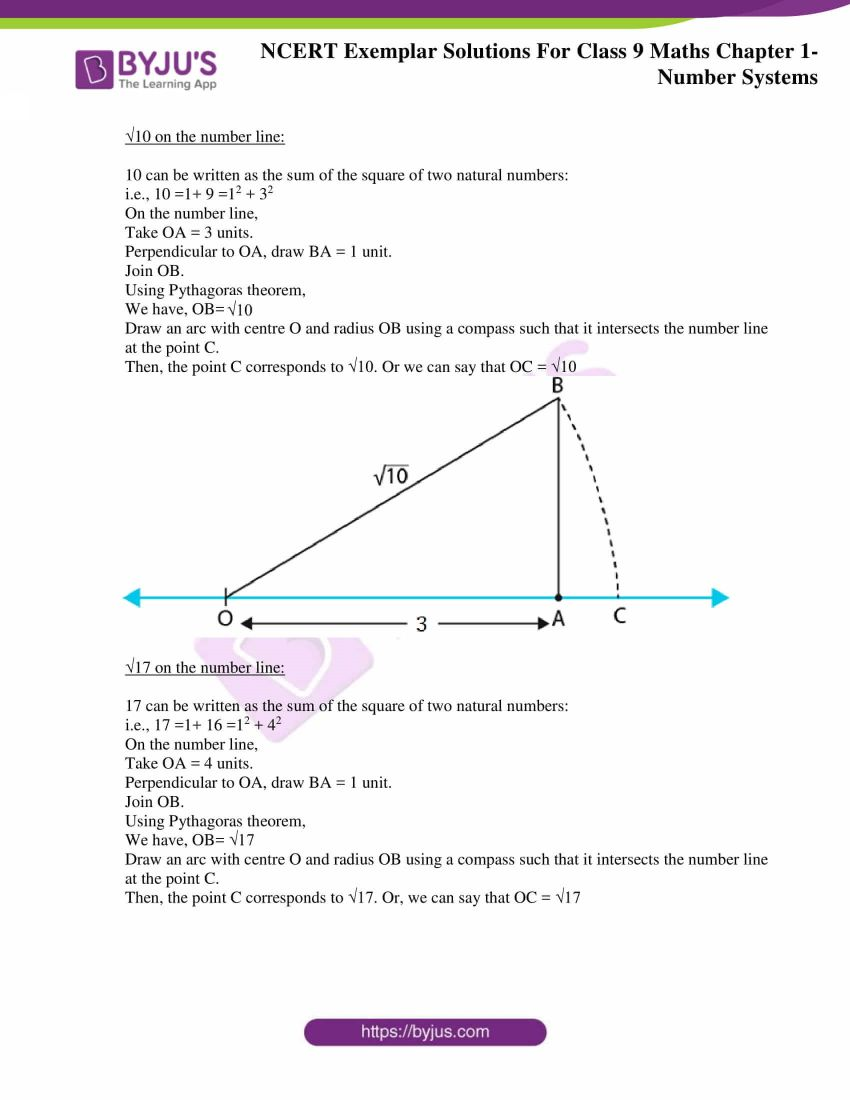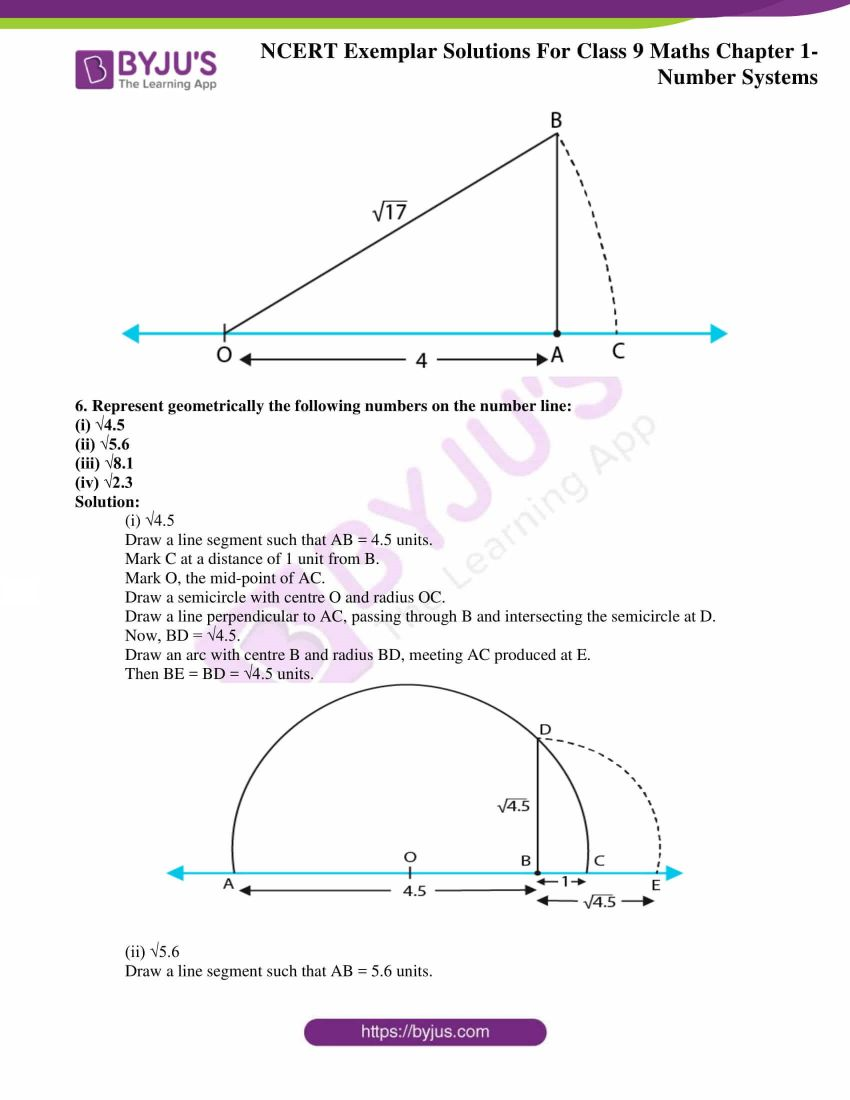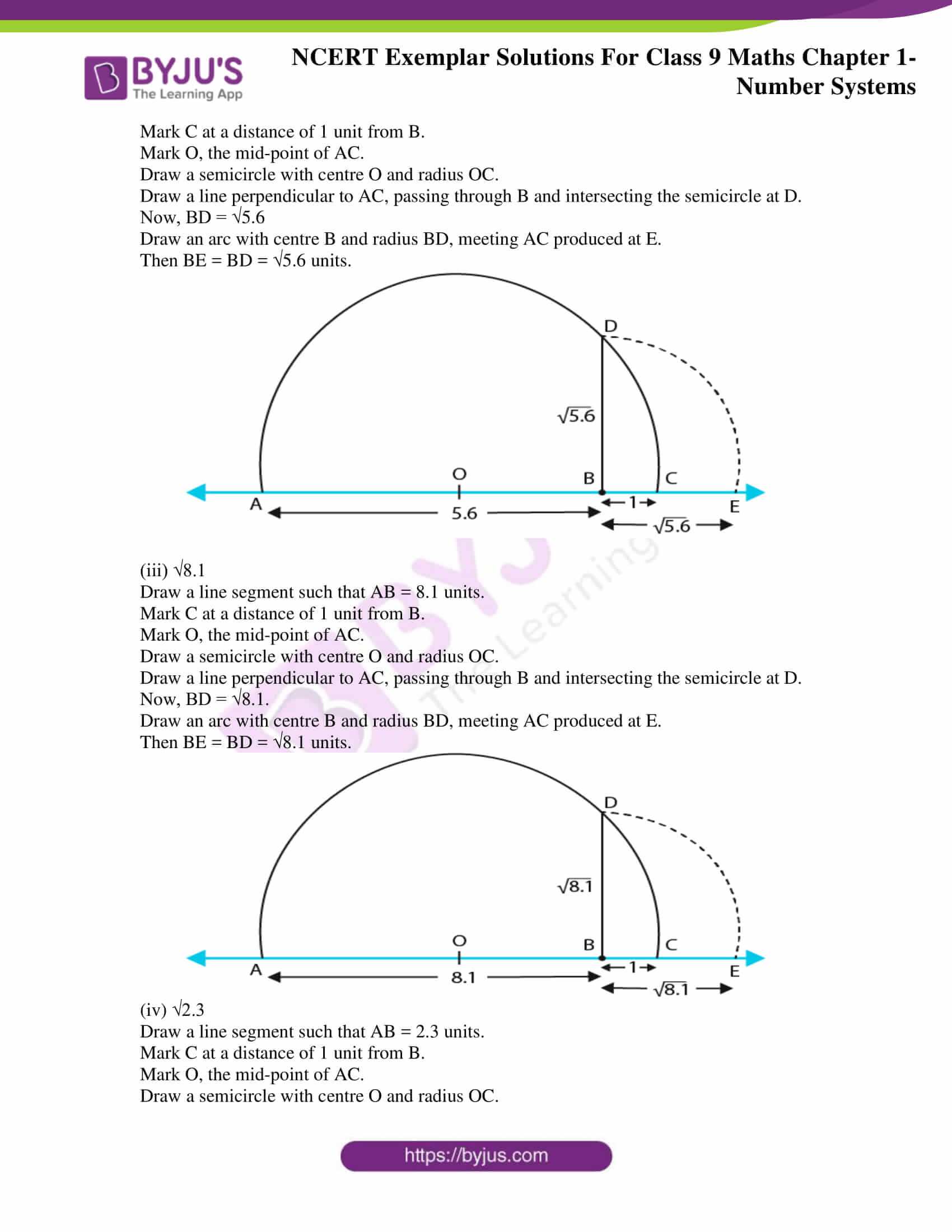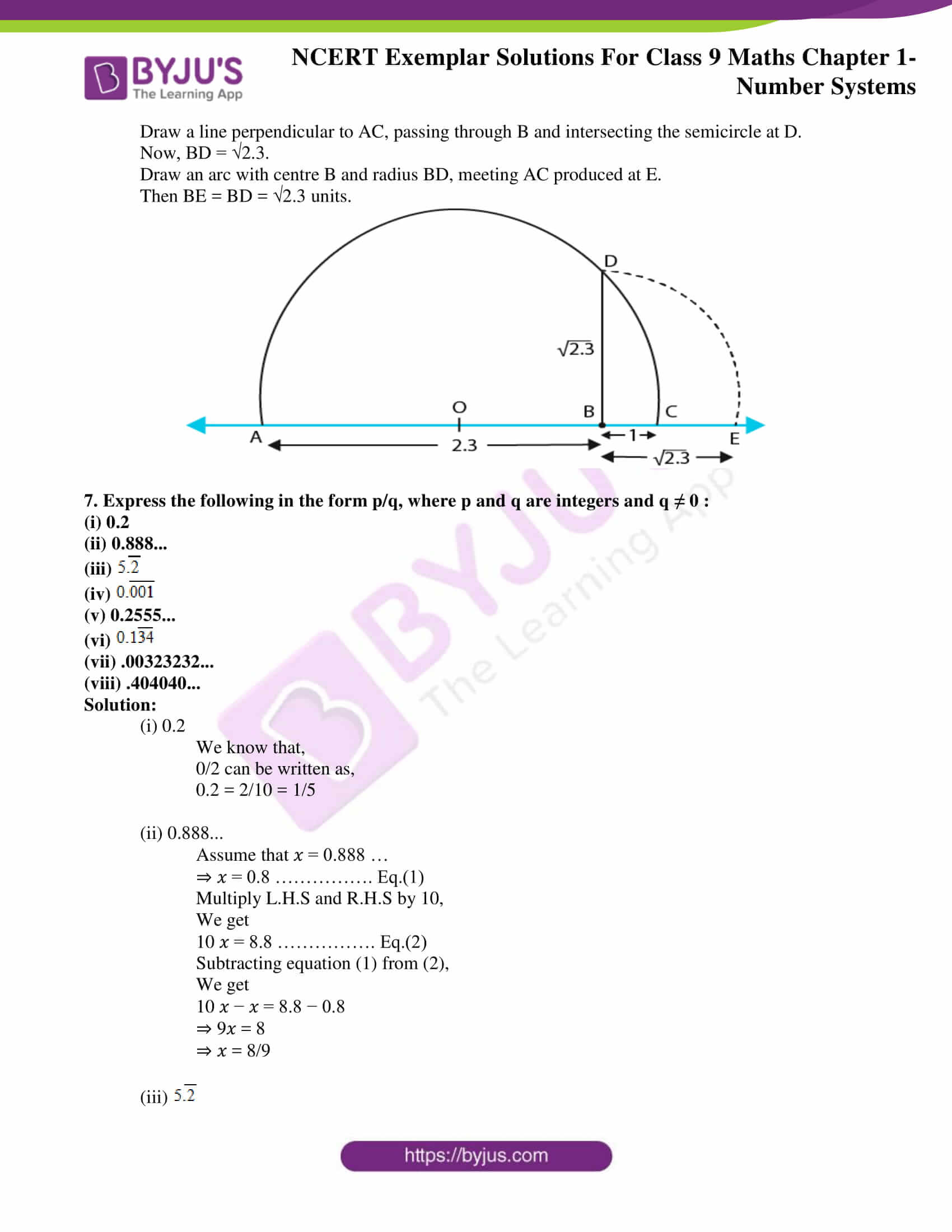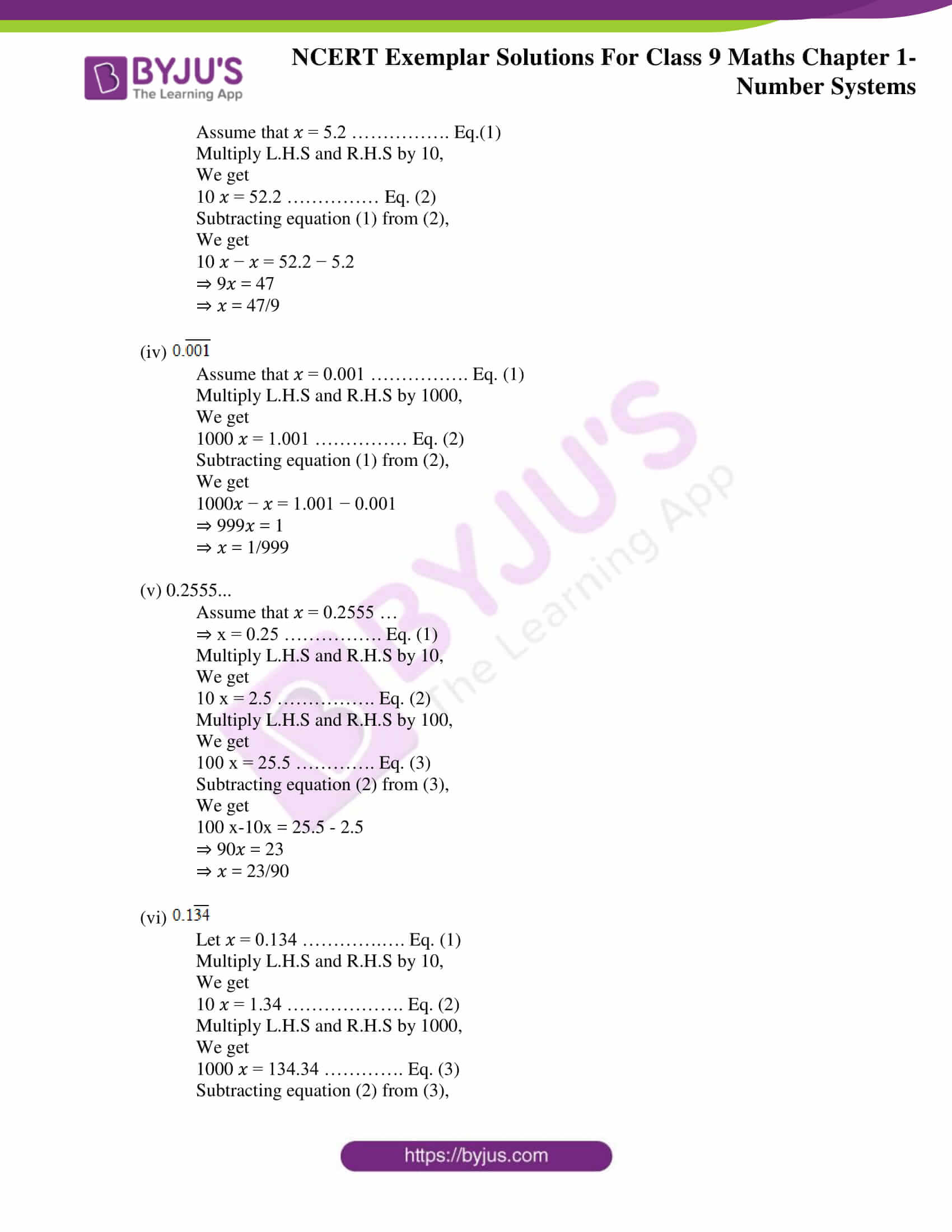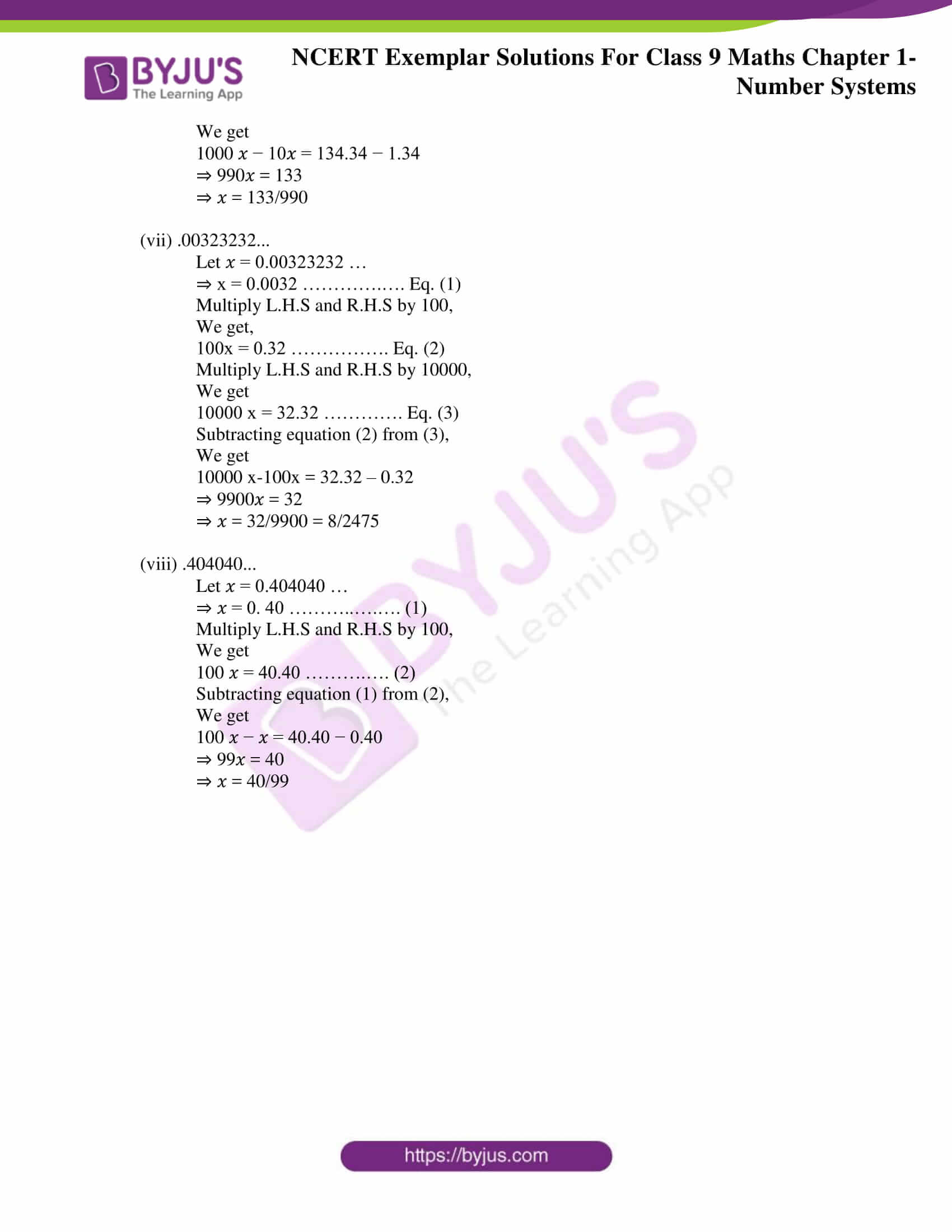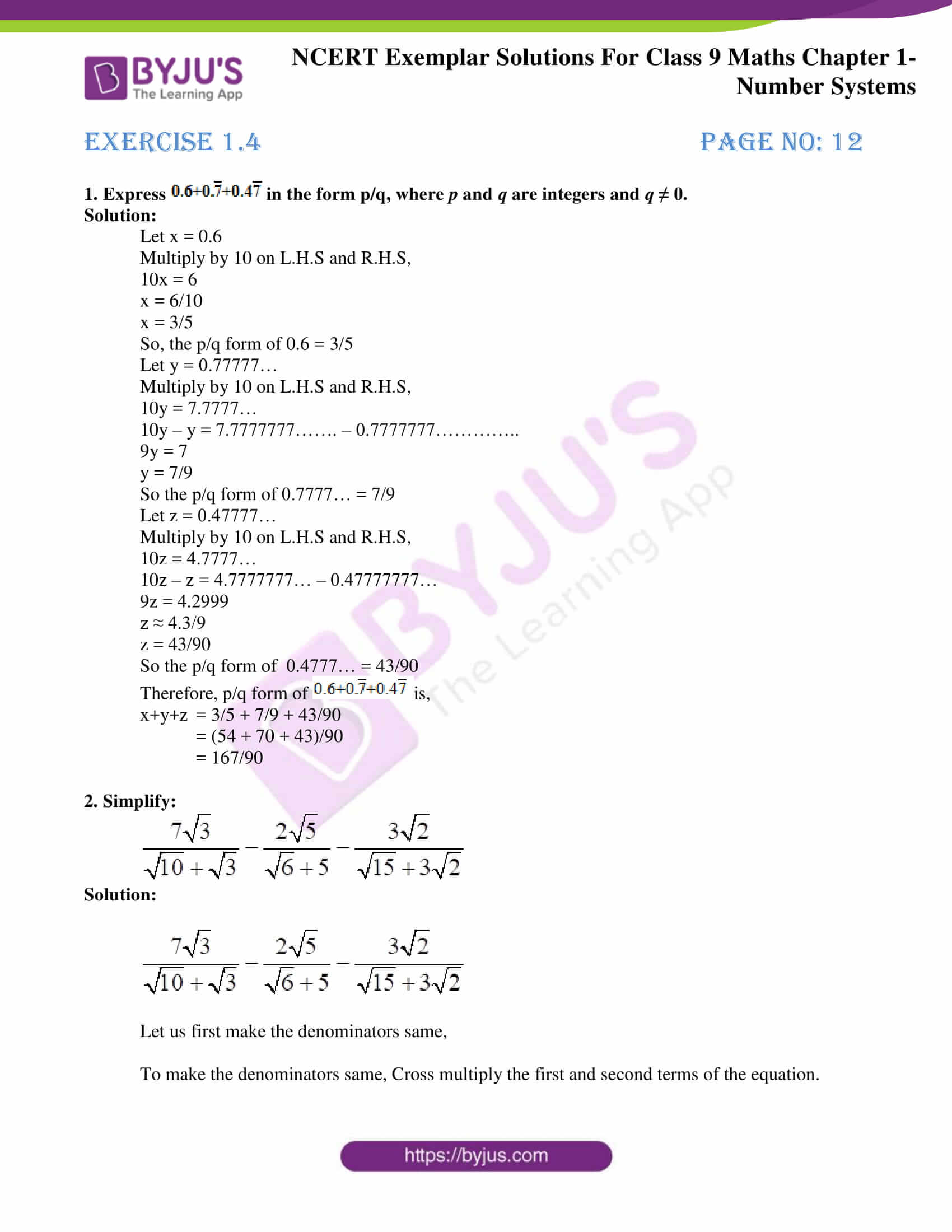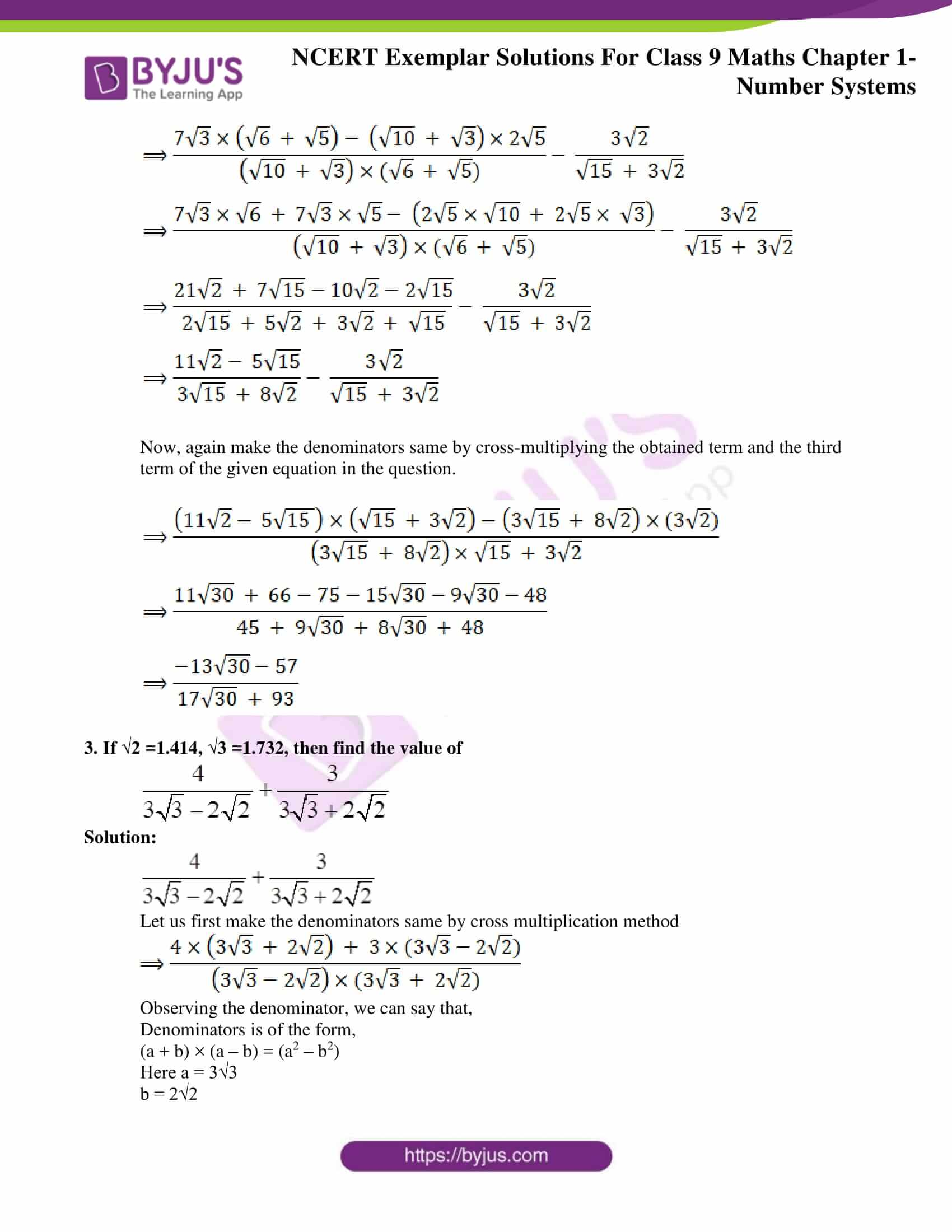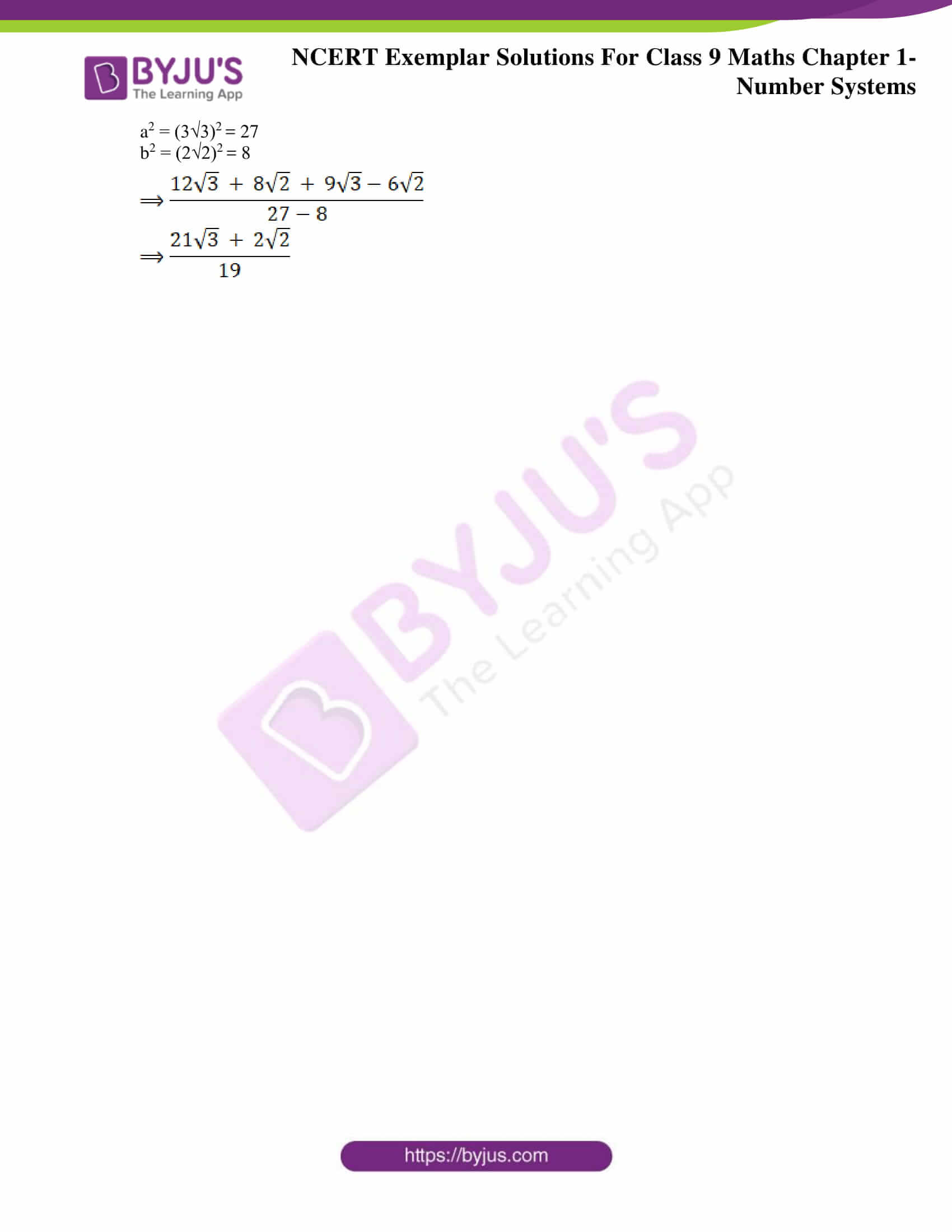### Access answers to NCERT Exemplar Solutions for Class 9 Maths Chapter 1 Number Systems

Exercise 1.1 Page No: 2

Write the correct answer in each of the following:

1. Every rational number is

(A) a natural number

(B) an integer

(C) a real number

(D) a whole number

Solution:

(C) a real number

Explanation:

We know that rational and irrational numbers taken together are known as real numbers. Therefore, every real number is either a rational number or an irrational number. Hence, every rational number is a real number.

Hence, (C) is the correct option.

2. Between two rational numbers

(A) there is no rational number

(B) there is exactly one rational number

(C) there are infinitely many rational numbers

(D) there are only rational numbers and no irrational numbers

Solution:

(C) there are infinitely many rational numbers

Explanation:

Between two rational numbers there are infinitely many rational number.

Hence, (C) is the correct option.

3. Decimal representation of a rational number cannot be

(A) terminating

(B) non-terminating

(C) non-terminating repeating

(D) non-terminating non-repeating

Solution:

(D) non-terminating non-repeating

Explanation:

The decimal representation of a rational number cannot be non-terminating and non- repeating.

Hence, (D) is the correct option

4. The product of any two irrational numbers is

(A) always an irrational number

(B) always a rational number

(C) always an integer

(D) sometimes rational, sometimes irrational

Solution:

(D) sometimes rational, sometimes irrational

Explanation:

The product of any two irrational numbers is sometimes rational and sometimes irrational.

Hence, (D) is the correct option

5. The decimal expansion of the number √2 is

(A) a finite decimal

(B) 1.41421

(C) non-terminating recurring

(D) non-terminating non-recurring

Solution:

(D) non-terminating non-recurring

Explanation:

The decimal expansion of the number √2 = 1.41421356237…

Hence, (D) is the correct option

6. Which of the following is irrational?

(A) √4/√9

(B) √12/√3

(C) √7

(D) √81

Solution:

(C) √7

Explanation:

(A) √4/√9 = 2/3

(B) √12/√3 = 2√3/√3 = 2

(C) √7 = 2.64575131106

(D) √81 = 9

Here, (C) √7 = 2.64575131106, is a non terminating decimal expansion.

Hence, (C) is the correct option

7. Which of the following is irrational?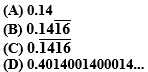Solution:

(D) 0.4014001400014…

Explanation:

A number is irrational if and only of its decimal representation is non-terminating and non-recurring.

(A) is a terminating decimal and therefore cannot be an irrational number.

(B) is a non-terminating and recurring decimal and therefore cannot be irrational.

(C) is a non-terminating and recurring decimal and therefore cannot be irrational.

(D) is a non-terminating and non-recurring decimal and therefore is an irrational number.

Hence, (D) is the correct option.

8. A rational number between √2 and √3 is

(A) (√2+√3)/2

(B) (√2. √3)/2

(C) 1.5

(D) 1.8

Solution:

(C) 1.5

Explanation:

√2 =1.4142135…. and √3 =1.732050807….

(A) (√2+√3)/2 = 1.57313218497… is a non-terminating and non-recurring decimal and therefore is an irrational number.

(B) (√2. √3)/2 = 1.22474487139… is a non-terminating and non-recurring decimal and therefore is an irrational number.

(C) 1.5 is a terminating decimal and therefore is a rational number.

(D) 1.8 is a terminating decimal and therefore is a rational number.

Here both 1.5 and 1.8 are rational numbers. But, 1.8 does not lie in between √2 =1.4142135…. and √3 =1.732050807…. Whereas 1.5 lies in between √2 =1.4142135…. and √3 =1.732050807….

Hence, (C) is the correct option.

9. The value of 1.999… in the form p/q, where p and q are integers and q ≠ 0 , is

(A) 19/10

(B) 1999/1000

(C) 2

(D) 1/9

Solution:

(C) 2

Explanation:

(A) 19/10 = 1.9

(B) 1999/1000= 1.999

(C) 2

(D) 1/9= 0.111….

Let x = 1.9999….. — ( 1 )

Multiply equation ( 1 ) with 10

10x = 19.9999….. — ( 2 )

Subtract equation (1) from equation(2) ,

We get,

9x = 18

x = 18 / 9

x = 2

Therefore,

x = 1.9999… = 2

Hence, (C) is the correct option.

10. 2√3 + √3 is equal to

(A) 2√6

(B) 6

(C) 3√3

(D) 4√6

Solution:

(C) 3√3

Explanation:

2√3 + √3

Taking √3 common,

We get,

√3(2+1) = √3(3) = 3√3

Hence, (C) is the correct option.

Exercise 1.2 Page No: 6

1. Let x and y be rational and irrational numbers, respectively. Is x + y necessarily an irrational number? Give an example in support of your answer.

Solution:

Yes, if x and y are rational and irrational numbers, respectively, then x+ y is an irrational number.

For example,

Let x = 5 and y = √2.

Then, x+y = 5 + √2 = 5 + 1.414… = 6.414…

Here, 6.414 is a non-terminating and non-recurring decimal and therefore is an irrational number.

Hence, x + y is an irrational number.

2. Let x be rational and y be irrational. Is xy necessarily irrational? Justify your answer by an example.

Solution:

No, if x is rational number and y is irrational number, then, xy is not necessarily an irrational number. It can be rational if x = 0, which is a rational number.

For Example:

Let y = √2, which is irrational.

Consider x = 2, which is rational.

Then, x × y = 2 × √2 = 2√2, which is irrational.

Consider x = 0, which is rational.

Then xy = 0 × √2 = 0, which is rational.

∴, we can conclude that, the product of a rational and an irrational number is always irrational, only if the rational number is not zero.

Exercise 1.3 Page No: 9

1. Find which of the variables x, y, z and u represent rational numbers and which irrational numbers:

(i) x2 = 5

(ii) y2 = 9
(iii) z2 = .04

(iv) 𝑢2 = 17/4

Solution:

(i) x2 = 5

On solving, we get

⇒ x = ± √5

Hence, x is an irrational number.

(ii) y2 = 9

On solving, we get

⇒ y = ± 3

Hence, y is a rational number.

(iii) z2 = .04

On solving, we get

⇒ z = ± 0.2

Hence, z is a rational number.

(iv) u2 = 17/4

On solving, we get

⇒ u = ± √17/2

√17 is irrational.

Hence, u is an irrational number

2. Find three rational numbers between

(i) –1 and –2

(ii) 0.1 and 0.11
(iii) 5/7 and 6/7

(iv) 1/4 and 1/5

Solution:

(i) –1 and –2

Three rational numbers between –1 and –2 are –1.1, –1.2 and –1.3.

(ii) 0.1 and 0.11

Three rational numbers between 0.1 and 0.11 are 0.101, 0.102 and 0.103.

(iii)5/7 and 6/7

5/7 can be written as (5 × 10)/(7 × 10) = 50/70

Similarly,

6/7 can be written as (6 × 10)/(7 × 10) = 60/70

Three rational numbers between 5/7 and 6/7 = three rational numbers between 50/70 and 60/70.

Three rational numbers between 5/7 and 6/7 are 51/70, 52/70, 53/70.

(iv)1/4 and 1/5

Here, according to the question,

LCM of 4 and 5 is 20.

Let us make the denominators common, 80.

(4 × 20) = 80 and (5 × 16) = 80

Hence,

1/4 can be written as (1 × 20)/(4 × 20) = 20/80

Similarly,

1/5 can be written as (1 × 16)/(5 × 16) = 16/80

Three rational numbers between 1/4 and 1/5 = three rational numbers between 16/80 and 20/80.

Therefore, the three rational numbers are 17/80, 18/80 and 19/80.

3. Insert a rational number and an irrational number between the following:

(i) 2 and 3

(ii) 0 and 0.1
(iii) 1/3 and 1/2

(iv) – 2/5 and 1/2
(v) 0.15 and 0.16

(vi) √2 and √3
(vii) 2.357 and 3.121
(viii) .0001 and .001
(ix) 3.623623 and 0.484848
(x) 6.375289 and 6.375738.

Solution:

(i) 2 and 3

So, rational number between 2 and 3 = 2.5

And, irrational number between 2 and 3 = 2.040040004…

(ii) 0 and 0.1

So, rational number between 0 and 0.1 = 0.05

And, irrational number between 0 and 0.1 = 0.007000700007…

(iii) 1/3 and 1/2

LCM of 3 and 2 is 6.

1/3 = 0.33

1/3 can be written as (1 × 20)/(3 × 20) = 20/60

½ = 0.5

1/2 can be written as (1 × 30)/(2 × 30) = 30/60

So, rational number between 1/3 and 1/2 = 25/60

And, irrational number between 1/3 and 1/2 = irrational number between 0.33 and 0.5 = 0.414114111…

(iv) – 2/5 and 1/2

LCM of 5 and 2 is 10.

-2/5 = -0.4

-2/5 can be written as (-2 × 2)/(5 × 2) = -4/10

1/2 = 0.5

1/2 can be written as (1 × 5)/(2 × 5) = 5/10

So, rational number between -2/5 and 1/2 = rational number between -4/10 and 5/10 = 1/10

And, irrational number between -2/5 and 1/2 = irrational number between -0.4 and 0.5 = 0.414114111…

(v) 0.15 and 0.16

Rational number between 0.15 and 0.16 = 0.151

Irrational number between 0.15 and 0.16 = 0.151551555…

(vi) √2 = 1.41 and √3 = 1.732

Rational number between √2 and √3 = rational number between 1.41 and 1.732 = 1.5

Irrational number between √2 and √3 = irrational number between 1.41 and 1.732 = 1.585585558…

(vii) 2.357 and 3.121

Rational number between 2.357 and 3.121 = 3

Irrational number between 2.357 and 3.121 = 3.101101110…

(viii) .0001 and .001

Rational number between .0001 and .001 = 0.00011

Irrational number between .0001 and .001 = 0.0001131331333…

(ix) 3.623623 and 0.484848

Rational number between 3.623623 and 0.484848 = 1

Irrational number between 3.623623 and 0.484848 = 1.909009000…

(x) 6.375289 and 6.375738.

Rational number between 6.375289 and 6.375738 = 6.3753

Irrational number between 6.375289 and 6.375738 = 6.375414114111…

4. Represent the following numbers on the number line:

7, 7.2, −3/2 , −12/5

Solution: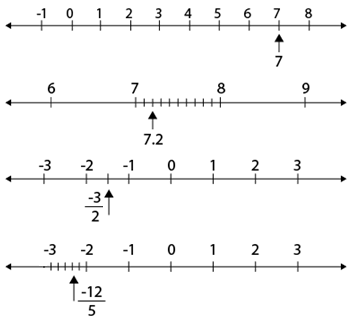5. Locate √5, √10 and √17 on the number line.

Solution:

√5 on the number line:

5 can be written as the sum of the square of two natural numbers:

i.e., 5 =1+ 4 =12 + 22

On the number line,

Take OA = 2 units.

Perpendicular to OA, draw BA = 1 unit.

Join OB.

Using Pythagoras theorem,

We have, OB= √5

Draw an arc with centre O and radius OB using a compass such that it intersects the number line at the point C.

Then, we get, C corresponds to √5. Or we can say that OC = √5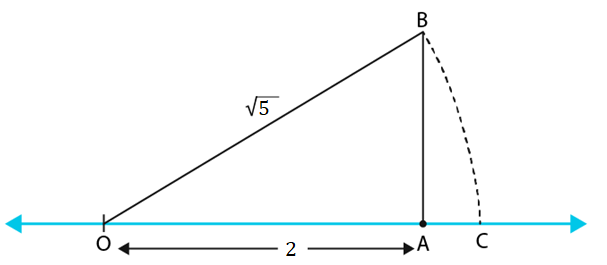√10 on the number line:

10 can be written as the sum of the square of two natural numbers:

i.e., 10 =1+ 9 =12 + 32

On the number line,

Take OA = 3 units.

Perpendicular to OA, draw BA = 1 unit.

Join OB.

Using Pythagoras theorem,

We have, OB= √10

Draw an arc with centre O and radius OB using a compass such that it intersects the number line at the point C.

Then, the point C corresponds to √10. Or we can say that OC = √10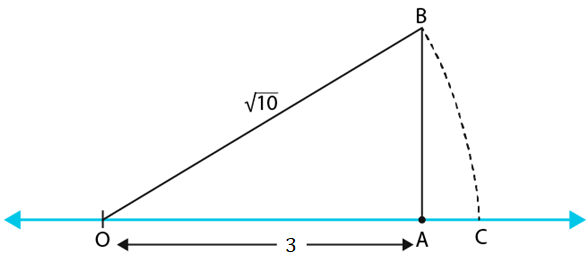√17 on the number line:

17 can be written as the sum of the square of two natural numbers:

i.e., 17 =1+ 16 =12 + 42

On the number line,

Take OA = 4 units.

Perpendicular to OA, draw BA = 1 unit.

Join OB.

Using Pythagoras theorem,

We have, OB= √17

Draw an arc with centre O and radius OB using a compass such that it intersects the number line at the point C.

Then, the point C corresponds to √17. Or, we can say that OC = √17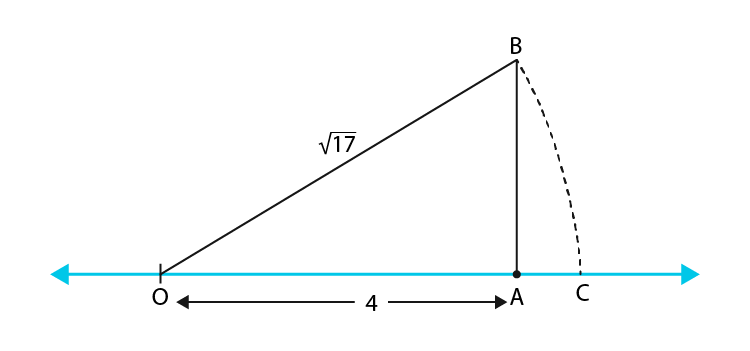6. Represent geometrically the following numbers on the number line:

(i) √4.5

(ii) √5.6

(iii) √8.1

(iv) √2.3

Solution:

(i) √4.5

Draw a line segment such that AB = 4.5 units.

Mark C at a distance of 1 unit from B.

Mark O, the mid-point of AC.

Draw a semicircle with centre O and radius OC.

Draw a line perpendicular to AC, passing through B and intersecting the semicircle at D.

Now, BD = √4.5.

Draw an arc with centre B and radius BD, meeting AC produced at E.

Then BE = BD = √4.5 units.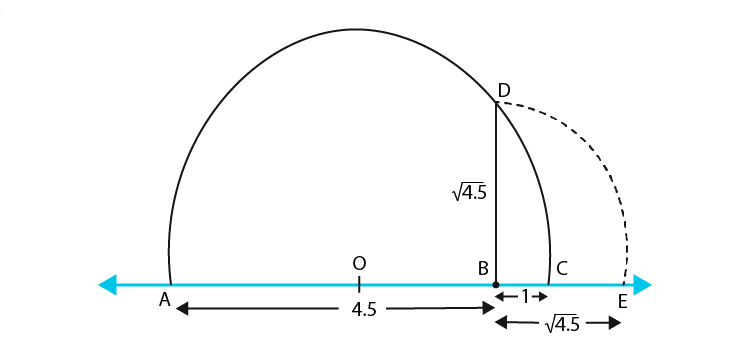(ii) √5.6

Draw a line segment such that AB = 5.6 units.

Mark C at a distance of 1 unit from B.

Mark O, the mid-point of AC.

Draw a semicircle with centre O and radius OC.

Draw a line perpendicular to AC, passing through B and intersecting the semicircle at D.

Now, BD = √5.6

Draw an arc with centre B and radius BD, meeting AC produced at E.

Then BE = BD = √5.6 units.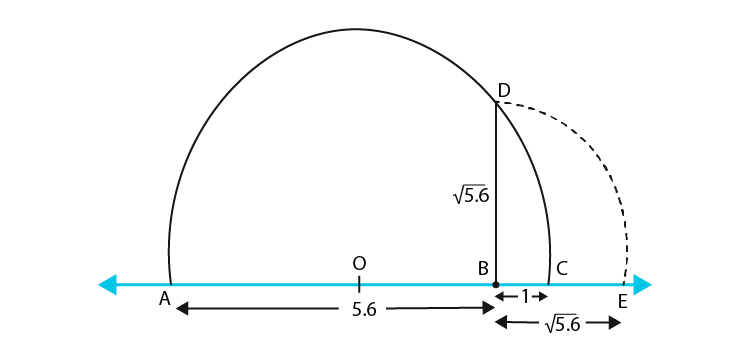(iii) √8.1

Draw a line segment such that AB = 8.1 units.

Mark C at a distance of 1 unit from B.

Mark O, the mid-point of AC.

Draw a semicircle with centre O and radius OC.

Draw a line perpendicular to AC, passing through B and intersecting the semicircle at D.

Now, BD = √8.1.

Draw an arc with centre B and radius BD, meeting AC produced at E.

Then BE = BD = √8.1 units.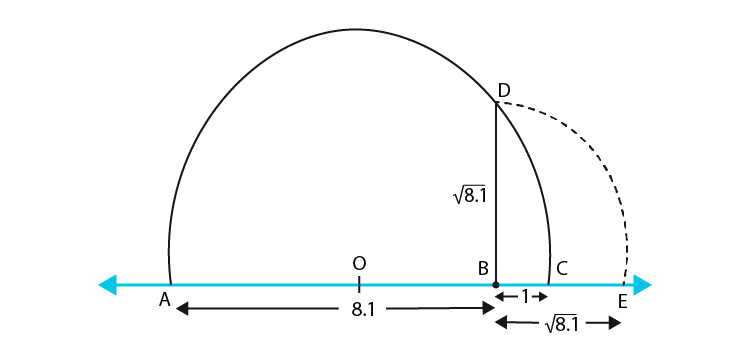(iv) √2.3

Draw a line segment such that AB = 2.3 units.

Mark C at a distance of 1 unit from B.

Mark O, the mid-point of AC.

Draw a semicircle with centre O and radius OC.

Draw a line perpendicular to AC, passing through B and intersecting the semicircle at D.

Now, BD = √2.3.

Draw an arc with centre B and radius BD, meeting AC produced at E.

Then BE = BD = √2.3 units.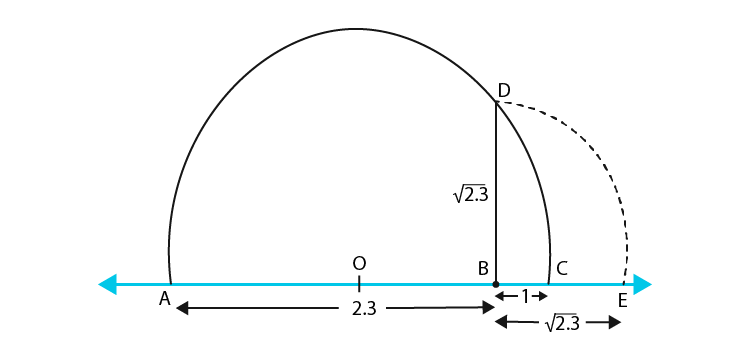7. Express the following in the form p/q, where p and q are integers and q ≠ 0 :

(i) 0.2

(ii) 0.888…

(iii)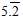(iv)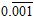(v) 0.2555…

(vi)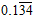(vii) .00323232…

(viii) .404040…

Solution:

(i) 0.2

We know that,

0/2 can be written as,

0.2 = 2/10 = 1/5

(ii) 0.888…

Assume that 𝑥 = 0.888 …

⇒ 𝑥 = 0.8 ……………. Eq.(1)

Multiply L.H.S and R.H.S by 10,

We get

10 𝑥 = 8.8 ……………. Eq.(2)

Subtracting equation (1) from (2),

We get

10 𝑥 − 𝑥 = 8.8 − 0.8

⇒ 9𝑥 = 8

⇒ 𝑥 = 8/9

(iii)Assume that 𝑥 = 5.2 ……………. Eq.(1)

Multiply L.H.S and R.H.S by 10,

We get

10 𝑥 = 52.2 …………… Eq. (2)

Subtracting equation (1) from (2),

We get

10 𝑥 − 𝑥 = 52.2 − 5.2

⇒ 9𝑥 = 47

⇒ 𝑥 = 47/9

(iv)Assume that 𝑥 = 0.001 ……………. Eq. (1)

Multiply L.H.S and R.H.S by 1000,

We get

1000 𝑥 = 1.001 …………… Eq. (2)

Subtracting equation (1) from (2),

We get

1000𝑥 − 𝑥 = 1.001 − 0.001

⇒ 999𝑥 = 1

⇒ 𝑥 = 1/999

(v) 0.2555…

Assume that 𝑥 = 0.2555 …

⇒ x = 0.25 ……………. Eq. (1)

Multiply L.H.S and R.H.S by 10,

We get

10 x = 2.5 ……………. Eq. (2)

Multiply L.H.S and R.H.S by 100,

We get

100 x = 25.5 …………. Eq. (3)

Subtracting equation (2) from (3),

We get

100 x-10x = 25.5 – 2.5

⇒ 90𝑥 = 23

⇒ 𝑥 = 23/90

(vi)Let 𝑥 = 0.134 ………….…. Eq. (1)

Multiply L.H.S and R.H.S by 10,

We get

10 𝑥 = 1.34 ………………. Eq. (2)

Multiply L.H.S and R.H.S by 1000,

We get

1000 𝑥 = 134.34 …………. Eq. (3)

Subtracting equation (2) from (3),

We get

1000 𝑥 − 10𝑥 = 134.34 − 1.34

⇒ 990𝑥 = 133

⇒ 𝑥 = 133/990

(vii) .00323232…

Let 𝑥 = 0.00323232 …

⇒ x = 0.0032 ………….…. Eq. (1)

Multiply L.H.S and R.H.S by 100,

We get,

100x = 0.32 ……………. Eq. (2)

Multiply L.H.S and R.H.S by 10000,

We get

10000 x = 32.32 …………. Eq. (3)

Subtracting equation (2) from (3),

We get

10000 x-100x = 32.32 – 0.32

⇒ 9900𝑥 = 32

⇒ 𝑥 = 32/9900 = 8/2475

(viii) .404040…

Let 𝑥 = 0.404040 …

⇒ 𝑥 = 0. 40 ………..….…. (1)

Multiply L.H.S and R.H.S by 100,

We get

100 𝑥 = 40.40 ……….…. (2)

Subtracting equation (1) from (2),

We get

100 𝑥 − 𝑥 = 40.40 − 0.40

⇒ 99𝑥 = 40

⇒ 𝑥 = 40/99

Exercise 1.4 Page No: 12

1. Express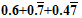in the form p/q, where p and q are integers and q ≠ 0.

Solution:

Let x = 0.6

Multiply by 10 on L.H.S and R.H.S,

10x = 6

x = 6/10

x = 3/5

So, the p/q form of 0.6 = 3/5

Let y = 0.77777…

Multiply by 10 on L.H.S and R.H.S,

10y = 7.7777…

10y – y = 7.7777777……. – 0.7777777…………..

9y = 7

y = 7/9

So the p/q form of 0.7777… = 7/9

Let z = 0.47777…

Multiply by 10 on L.H.S and R.H.S,

10z = 4.7777…

10z – z = 4.7777777… – 0.47777777…

9z = 4.2999

z ≈ 4.3/9

z = 43/90

So the p/q form of  0.4777… = 43/90

Therefore, p/q form of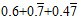is,

x+y+z = 3/5 + 7/9 + 43/90

= (54 + 70 + 43)/90

= 167/90

2. Simplify: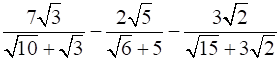Solution:Let us first make the denominators same,

To make the denominators same, Cross multiply the first and second terms of the equation.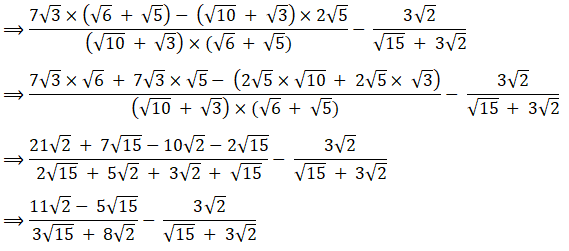Now, again make the denominators same by cross-multiplying the obtained term and the third term of the given equation in the question.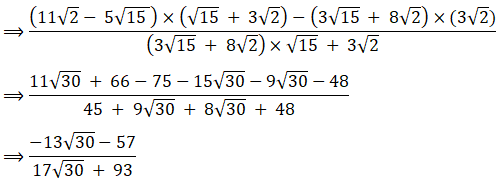3. If √2 =1.414, √3 =1.732, then find the value of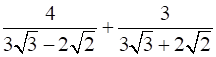Solution:Let us first make the denominators same by cross multiplication method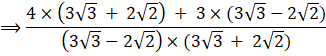Observing the denominator, we can say that,

Denominators is of the form,

(a + b) × (a – b) = (a2 – b2)

Here a = 3√3

b = 2√2

a2 = (3√3)2 = 27

b2 = (2√2)2 = 8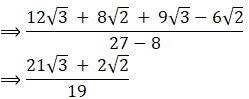There are some other sources of learning provided by BYJU’S for students such as notes, exemplar books, NCERT Solutions and question papers to prepare for their exams and score good marks. Also, it is recommended to solve sample papers and previous year question papers which gives an idea of question types asked in the CBSE exam from Chapter 1 Number System. Solving the NCERT Exemplar questions will help students to understand the chapter and to practice higher-level questions related to the number system.

Download BYJU’S-The Learning App and get personalized videos, explaining different types of Maths topics such as number systems and different types of numbers, etc. and experience a new way of learning to understand the concepts easily.

## Frequently Asked Questions on NCERT Exemplar Solutions for Class 9 Maths Chapter 1

### What are the topics covered in Chapter 1 of NCERT Exemplar Solutions for Class 9 Maths?

The topics covered in Chapter 1 of NCERT Exemplar Solutions for Class 9 Maths are:
1. Rational Numbers and Irrational numbers
2. Finding rational numbers between two given numbers
3. Locating irrational numbers in a number line
4. Real Numbers and their Decimal Expansions are terminating or non-terminating, recurring or non-recurring.
5. Finding irrational numbers between two given numbers
6. Operations performed on real numbers
7. Rationalizing the denominator
8. Laws of Exponents for Real Numbers

### What are rational numbers according to NCERT Exemplar Solutions for Class 9 Maths Chapter 1?

Rational numbers are numbers which can be expressed as a fraction and also as positive numbers, negative numbers and zero. It can be written as p/q, where q is not equal to zero. The word rational is derived from the word ‘ratio’, which actually means a comparison of two or more values or integer numbers and is known as a fraction. In simple words, it is the ratio of two integers.
Example: 3/2 is a rational number. It means integer 3 is divided by another integer 2.

### How to Rationalize the denominator discussed in Chapter 1 of NCERT Exemplar Solutions for Class 9 Maths?

In Mathematics, we rationalise the denominator, when the given fraction contains a radical term or a surd in the denominator. These radical terms include square root and cube root. To rationalise the denominator with square root, multiply and divide the given fraction with the same square root value. This way, the denominator will be a rational number.